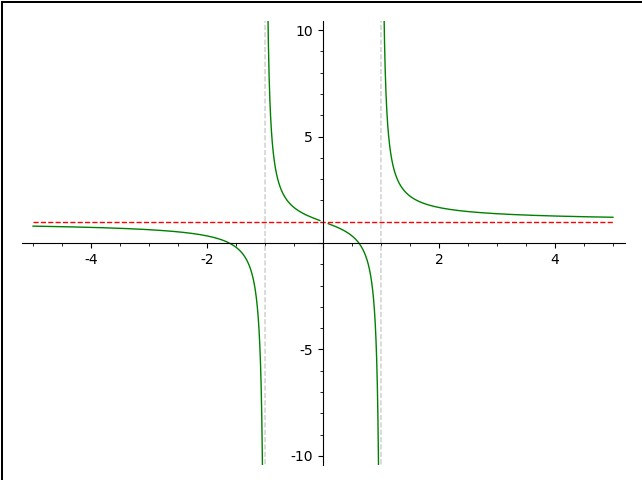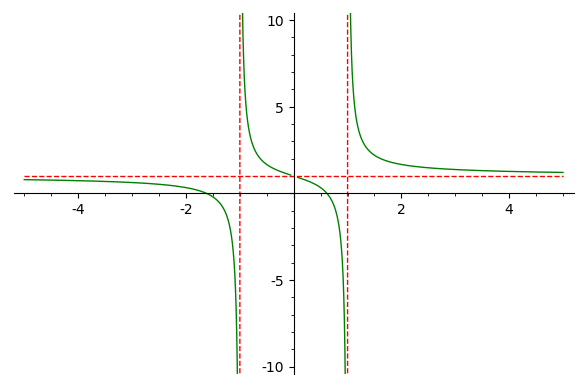# How do I plot Asymptotes of a curve

I wanna know the codes for plotting Asymptotes of any curve . I'll be so greatfull if anyone can help me with examples

edit retag close merge delete

Could you give us an example of a curve you are trying to plot? There is an extensive documentation.

(3x^2-2x+1)/(x-1) I want slant asymptotes for this equation

Sort by » oldest newest most voted

Here's an example to illustrate some of the documentation:

f=x/(x^2-1)+1
p1 = plot(f,(-5,5), detect_poles='show',color='green', ymin=-10,ymax=10)
p2 = plot(1,(-5,5), color='red', linestyle='--')
show(p1+p2)


Run the code in a Sage Cell Server and you'll get:The vertical asymptotes are done automatically with detect_poles='show' in plotting f. The result is put into p1. Since vertical asymptotes involve the function going off to plus or minus infinity, ymin=-10,ymax=10 sets the values to which the plot will go. The horizontal asymptotes are something you need to find; of course, SAGE can help. There is one horizontal asymptote and I've put its plot in p2. Finally, I combine the 2 using show()

moreThis is a follow up of @dazedANDonfused's answer to show how to plot all the asymptotes with the same style, that is, how to get the following figure:This can be achieved with the following modification of @dazedANDonfused's code:

f = x/(x^2-1)+1
p1 = plot(f,(-5,5), detect_poles='show',color='green', ymin=-10,ymax=10)
p2 = plot(1,(-5,5), color='red', linestyle='--')

for curve in p1:
if len(curve)==2:
opt = curve.options()
opt["rgbcolor"] = "red"
curve.set_options(opt)

show(p1+p2)


Please, note that p1 contains several lines (the vertical asymptotes and four arcs of the curve $y=f(x)$), each one having its own style and number of points. It can be seen as follows:

sage: print(p1)
Graphics object consisting of 6 graphics primitives
sage: for curve in p1:
....:     print(curve)
Line defined by 233 points
Line defined by 2 points
Line defined by 162 points
Line defined by 152 points
Line defined by 2 points
Line defined by 214 points


Asymptotes are the only lines with two points. The loop added to @dazedANDonfused's code selects these lines and changes the item corresponding to color in the dictionary which contains the line options.

more

That's nice! I wasn't aware of how you could change the asymptote color.